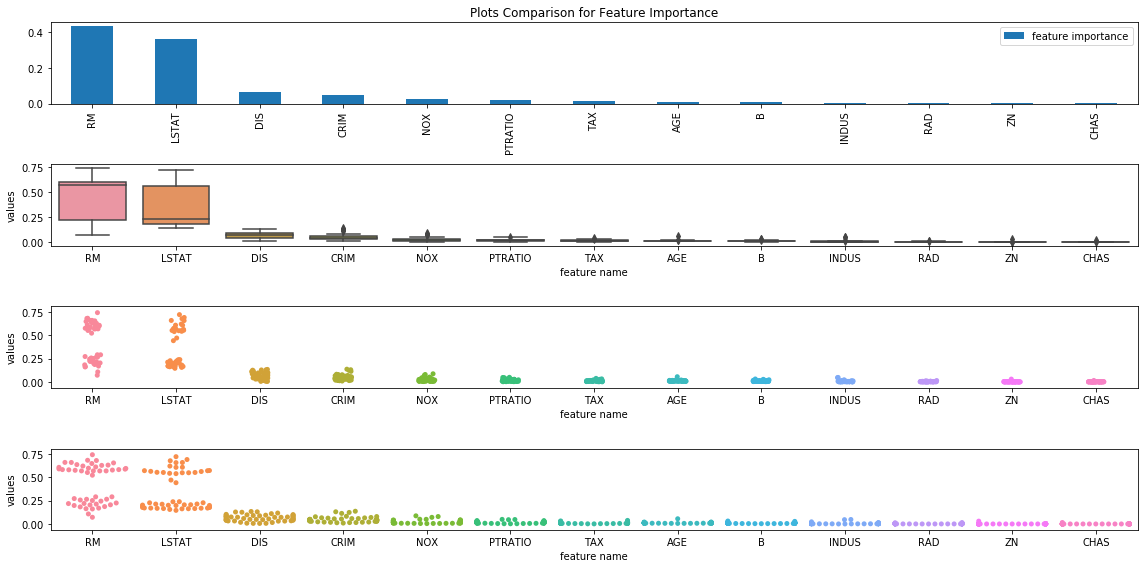# Feature Importance

## Goal¶

This post aims to introduce how to obtain feature importance using random forest and visualize it in a different format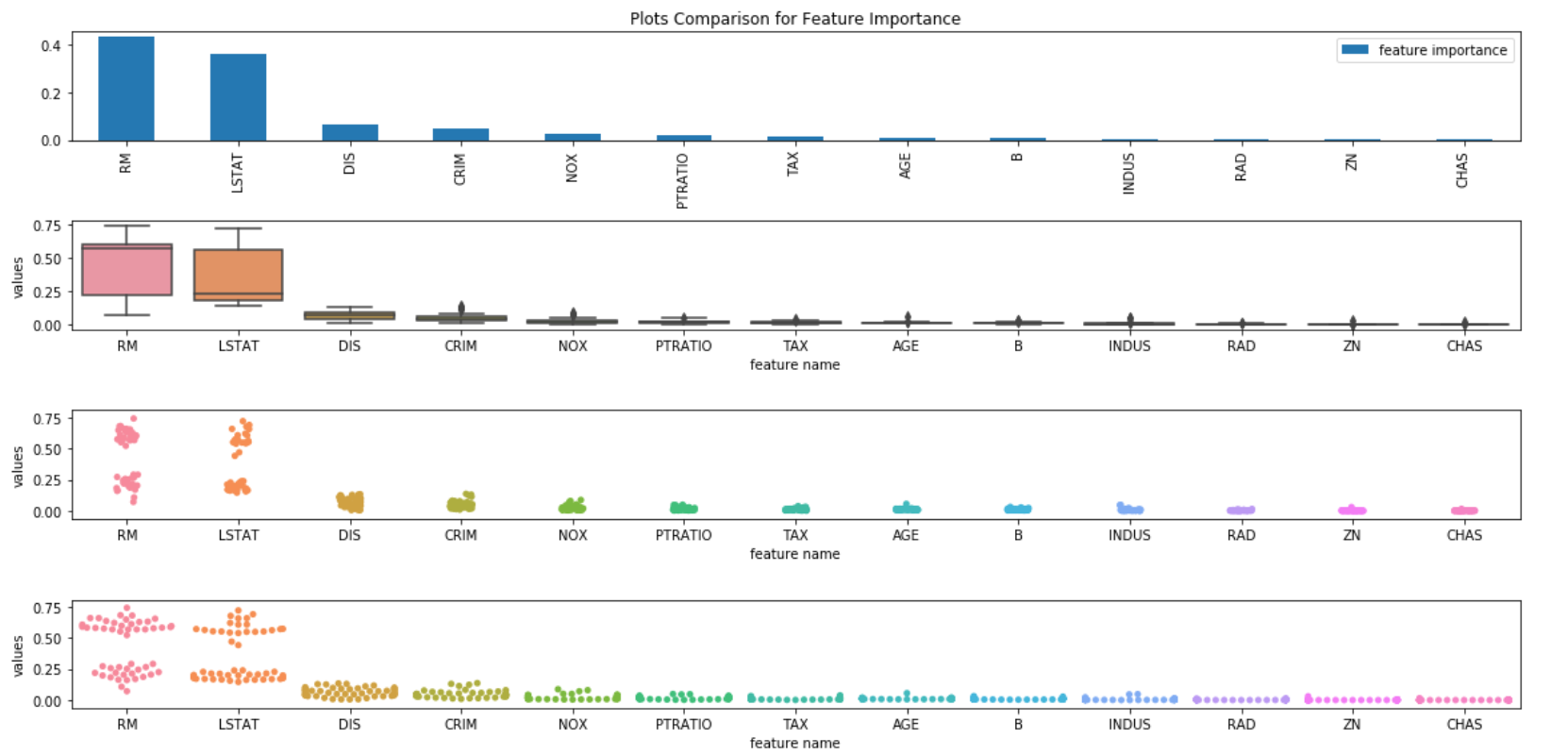Reference

## Libraries¶

In :
import pandas as pd
import numpy as np
from sklearn.model_selection import cross_val_score
from sklearn.ensemble import RandomForestRegressor
import matplotlib.pyplot as plt
import seaborn as sns
%matplotlib inline


## Configuration¶

In :
import matplotlib as mpl
mpl.rcParams['figure.figsize'] = (16, 6)


In :
boston = load_boston()

df_boston = pd.DataFrame(data=boston.data, columns=boston.feature_names)

Out:
CRIM ZN INDUS CHAS NOX RM AGE DIS RAD TAX PTRATIO B LSTAT
0 0.00632 18.0 2.31 0.0 0.538 6.575 65.2 4.0900 1.0 296.0 15.3 396.90 4.98
1 0.02731 0.0 7.07 0.0 0.469 6.421 78.9 4.9671 2.0 242.0 17.8 396.90 9.14
2 0.02729 0.0 7.07 0.0 0.469 7.185 61.1 4.9671 2.0 242.0 17.8 392.83 4.03
3 0.03237 0.0 2.18 0.0 0.458 6.998 45.8 6.0622 3.0 222.0 18.7 394.63 2.94
4 0.06905 0.0 2.18 0.0 0.458 7.147 54.2 6.0622 3.0 222.0 18.7 396.90 5.33

## Train a Random Forest Regressor¶

In :
reg = RandomForestRegressor(n_estimators=50)
reg.fit(df_boston, boston.target)

Out:
RandomForestRegressor(bootstrap=True, criterion='mse', max_depth=None,
max_features='auto', max_leaf_nodes=None,
min_impurity_decrease=0.0, min_impurity_split=None,
min_samples_leaf=1, min_samples_split=2,
min_weight_fraction_leaf=0.0, n_estimators=50, n_jobs=None,
oob_score=False, random_state=None, verbose=0, warm_start=False)

## Obtain feature importance¶

### average feature importance¶

In :
df_feature_importance = pd.DataFrame(reg.feature_importances_, index=boston.feature_names, columns=['feature importance']).sort_values('feature importance', ascending=False)
df_feature_importance

Out:
feature importance
RM 0.434691
LSTAT 0.362675
DIS 0.065282
CRIM 0.048311
NOX 0.024685
PTRATIO 0.018163
TAX 0.012388
AGE 0.011825
B 0.010220
INDUS 0.006348
ZN 0.001503
CHAS 0.000950

### all feature importance for each tree¶

In :
df_feature_all = pd.DataFrame([tree.feature_importances_ for tree in reg.estimators_], columns=boston.feature_names)

Out:
CRIM ZN INDUS CHAS NOX RM AGE DIS RAD TAX PTRATIO B LSTAT
0 0.014397 0.000270 0.000067 0.001098 0.030470 0.160704 0.005805 0.040896 0.000915 0.009357 0.006712 0.008223 0.721085
1 0.027748 0.000151 0.004632 0.000844 0.079595 0.290730 0.020392 0.055907 0.012544 0.011589 0.018765 0.006700 0.470404
2 0.082172 0.000353 0.003930 0.002729 0.009873 0.182772 0.009487 0.053868 0.002023 0.014475 0.025605 0.004799 0.607914
3 0.020085 0.000592 0.006886 0.001462 0.016882 0.290993 0.007097 0.074538 0.001960 0.003679 0.012879 0.011265 0.551682
4 0.012873 0.001554 0.003002 0.000521 0.013372 0.251145 0.010757 0.110498 0.002889 0.007838 0.009357 0.027501 0.548694
In :
# Melted data i.e., long format
df_feature_long = pd.melt(df_feature_all,var_name='feature name', value_name='values')


## Visualize feature importance¶

The feature importance is visualized in the following format:

• Bar chart
• Box Plot
• Strip Plot
• Swarm Plot
• Factor plot

### Bar chart¶

In :
df_feature_importance.plot(kind='bar');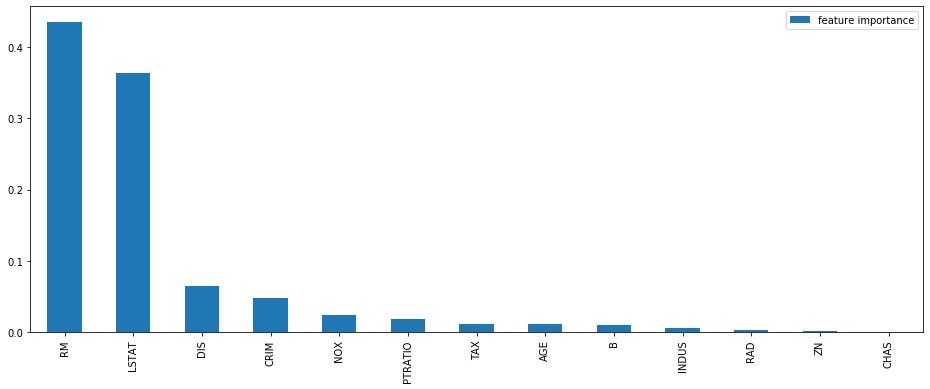### Box plot¶

In :
sns.boxplot(x="feature name", y="values", data=df_feature_long, order=df_feature_importance.index);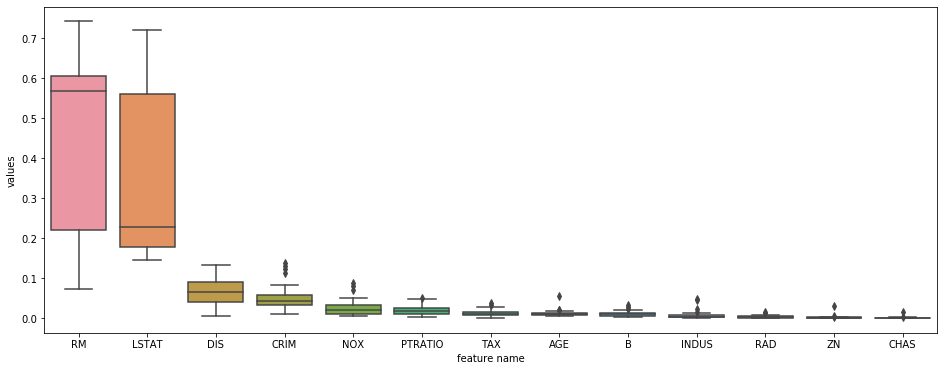### Strip Plot¶

In :
sns.stripplot(x="feature name", y="values", data=df_feature_long, order=df_feature_importance.index);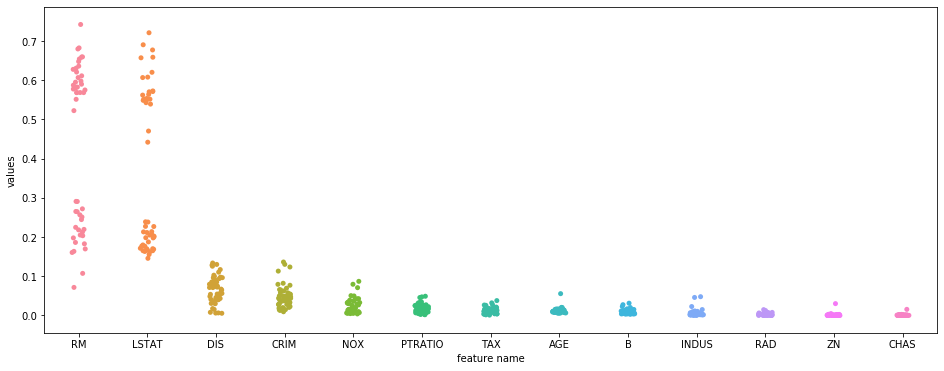### Swarm plot¶

In :
sns.swarmplot(x="feature name", y="values", data=df_feature_long, order=df_feature_importance.index);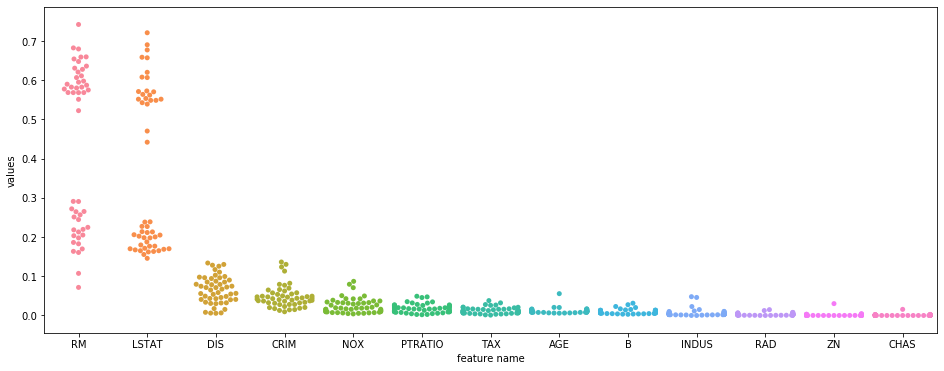### All¶

In :
fig, axes = plt.subplots(4, 1, figsize=(16, 8))
df_feature_importance.plot(kind='bar', ax=axes, title='Plots Comparison for Feature Importance');
sns.boxplot(ax=axes, x="feature name", y="values", data=df_feature_long, order=df_feature_importance.index);
sns.stripplot(ax=axes, x="feature name", y="values", data=df_feature_long, order=df_feature_importance.index);
sns.swarmplot(ax=axes, x="feature name", y="values", data=df_feature_long, order=df_feature_importance.index);
plt.tight_layout()